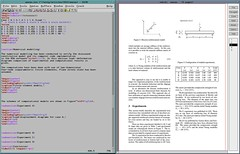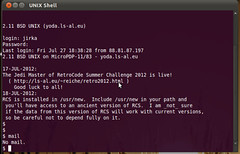# No shocking news

I use non-SGI computers more and more (both my phone and my tablet are Ubuntu Touch based and my 2nd desktop is the Intel Compute Stick with the Ubuntu) but I still use my O2 most time.

The O2 has a bunch of programs on all virtual desktops (some manuals, text editors JabRef and so). There are visible only some terminals (RXVT ones) which are connected to remote machines (they are much faster than my O2 so I use them for actual number crunching). The Vim and the Gnuplot are of course running locally…

# Current desktop

After several years I started to have lectures in an one of our basic courses. It means preparation and updating of presentations in the LaTeX with images created in the XFig. So my desktop looks like this the most of time:Well, this is only an illustration as the edited texts are different…

# BASIC on Zaurus

The Sharp Zaurus can be also used as a development machine for BASIC programming. The Bywater BASIC Interpreter can be easily compiled here and it of course runs fast. Both Vim and ZEditor can be used to edit program files (but there is no usefull source code highlighting, unfortunately).

I have tried to run a Gauss solver for a symmetric, positive definite system of linear equations. A full, dense left-side matrix is assumed (actually, only the upper triangle of the matrix is stored).

By the way, an exactly the same code runs flawlesly on the Elektronika MK-90 handheld computer (and thus it may run on the DVK serie of Soviet computers, too). It’s a little bit advanced version of the program that was presented there before some time.

```REM Gauss Elimination (full, dense matrix A)
REM Indexes start from 1
REM Only symmetric upper part of matrix is stored and used
REM Uses 1D arrays
REM "A" Indexes: L = I +(J*J-J)/2 -1

REM Data input:
10 DATA 3 1 2 3  2 1 3 3 3 7 5 5 5
30 DIM A(6)
35 DIM B(3)
40 FOR I = 1 TO N
50 FOR J = 1 TO N
55 IF J >= I THEN
58 LET L = I +(J*J-J)/2 -1
65 ELSE
67 END IF
70 NEXT J
80 NEXT I
90 FOR I = 0 TO N-1 STEP 1
100 NEXT I

REM Control print of data:
140 FOR I = 1 TO N
150 FOR J = 1 TO N
155 LET L = I +(J*J-J)/2
160 PRINT A(L);
170 NEXT J
175 PRINT " |";B(I-1)
180 NEXT I

REM Forward run:
300 FOR K = 1 TO N-1
310 FOR I = K+1 TO N
311 LET L = K + (I*I-I)/2 -1
312 LET M = K + (K*K-K)/2 -1
320 LET C = A(L)/A(M)
330 FOR J = I TO N
331 LET L = I +(J*J-J)/2 -1
332 LET M = K +(J*J-J)/2 -1
340 LET A(L) = A(L) - C*A(M)
350 NEXT J
360 LET B(I-1) = B(I-1) - C*B(K-1)
370 NEXT I
380 NEXT K
385 LET L = N +(N*N-N)/2 -1
390 LET B(N-1) = B(N-1) / A(L)

REM Backward run:
400 FOR I = N-1 TO 1 STEP -1
410 LET S = 0
420 FOR J = I+1 TO N
421 LET L = I +(J*J-J)/2 -1
430 LET S = S + A(L) * B(J-1)
440 NEXT J
441 LET L = I +(I*I-I)/2 -1
450 LET B(I-1) = (B(I-1)-S)/A(L)
460 NEXT I

REM Results:
500 PRINT "Results:"
510 FOR I = 0 TO N-1
520 PRINT B(I)
530 NEXT I
```

The input data hare hard-coded in the DATA statement, sorry for that. The first value is number of equations, followed by the matrix data (row by row) and the the right-hand vector is located.

# Sun Ultra 20

I still have a Ultra 20 workstation from Sun Microsystems. I have got it in 2005 (shortly after its introduction) and I used it for some computations. Then it was used by my wife and but it was replaced by her X60 laptop (the laptop is much more quiet and consumes less energy). There also were some hardware issues – I had to replace the graphics board twice. I also didn’t used this computer because I like my O2 much more. It’s comparably noisy and much slower but it has lower power consumption and runs IRIX (among other cool features). Thus the poor Ultra 20 wasn’t actually used for about half of its life.Technically, the coolest part of the Ultra 20 is the big “Sun Microsystems” logo on the case and the BIOS welcome screen. The rest is less or more ordinary PC. It is an extremelly well designed and build but still it is a PC with a server mainboard (the Tyan Tiger one) and with AMD Opteron processor.

But before some time I was stuck with problem which is too big to be solved on my other machines (2.5 GB of RAM and a decent processor is required). The O2 has too small memory for that (not speaking about its 250 MHz CPU). A decent dual-processor SGI like Octane or small Origin 200/300 might be a possible solution but I still don’t have working one. My Lenovo laptop has also too litle memory and also a low-power (read: slow) CPU. I also thought about use my G5 iMac for this task. But its upgrade (there is only 768 MB of RAM at the moment) looked to be too expensive. So it preservedit’s role of the only allowed computer in our living room where it serves as a DVD/media player…

Thus I returned to the good old Ultra 20. I had to replace the GFX board (there was a pasivelly cooled Quadro 290, now there is something similar but with active cooler) and a CMOS battery. Then I added some memory (there was 512 MB from the factory, I later added a 2 GB and now I replaced everything with 4x1GB modules). So now I have a box with 4 GB of RAM and with a dual-core 2.2 GHz Opteron (it was upgraded from single-core 1.8 GHz a few years after I got the system). The price of the upgrade was very low (the GFX board was taken from an non-working machine and the memory cost noly a few bucks).So I have returned my Ultra 20 to life. It’s only for a specific task and probably for a short period of time. It’s not as cool as Logout’s PowerPC/POWER desktops but it helped me a lot. For those who are curious about the operating system: it’s Ubuntu 12.04 with MATE desktop. It was installed because of my wife (the first Ubuntu here was Hardy Heron, I think) and I’m too lazy to replace it with something different. At the moment I don’t see a reason to replace it because it work for me. It’s a workstation not a playstation. 😉

# Casio FX-700P programming

I decided to make something usefull for my FX-700P. So I wrote a program that gives internal forces from a beam and computes necessary cross-section (for steel beams and the IPE-type cross sections only). The program uses several simplifications, it takes into account only a bending moments so it is for a preliminary design only. I actually needed it for some work in the wild when no real computer with me and when a fast (and thus just a preliminary) decision is needed.

So the program takes internal forces (bending moment, shear force and normal force) and uses basic computations (normal stress from M/W+N/A and a shear stress computation) to decide what IPE size is enough.
The IPE cross section parameters are approximated by continuous functions to save the memory of the calculator.

The code for the FX-700P looks like this:

```10  INPUT "M=",M
20  INPUT "V=",V
30  INPUT "N=",N
40  D = 235000000 * 0.9
100 J = 0 TO 12 STEP 1
110 H = 80 + J*20
120 A = H*H*0.000338738+H*0.0836899 -1.60321
130 I = H*H*H*0.000475247 -0.0512346*H*H + 212.463
140 B = 0.0148498*H + 2.62096
150 F = 0.0249428*H + 3.39449
160 E = 0.465021*H + 8.23748
170 S = (F*B*H/2)/1000000+((H/2.0-B/2.0)*F)/1000000
180 H = H/1000
190 A = A/10000
200 I = I/100000000
210 W = I/(0.5*h)
300 IF ABS(N/A - M/W) > D THEN 400
310 IF ABS(N/A + M/W) > D THEN 400
320 IF ABS(V*S / I*B) > D THEN 400
330 GOTO 410
400 NEXT J
410 PRINT "IPE";H*1000
```

For a comparison there is a C code that does exactly the same:

```int assess_IPE(double M, double V, double N)
{
int i ;
double h, A, I, W, S, Sh,f,e,a, fy;
double sigma_top, sigma_bot, tau_top, tau_max ;

fy = 235e6 * 0.9 ; /* design value of yield stress */

for (i=0; i 240)&&(h = 300) h = 330 ; /* fix fo real h sizes */

/* approximations: */
A = h*h*0.000338738  + h*0.0836899 -1.60321 ;
I = h*h*h*0.000475247 -0.0512346*h*h + 212.463 ;
a = 0.0148498*h + 2.62096;  /* wall thickness */
f = 0.0249428*h + 3.39449 ; /* thicknes of top part  */
e = 0.465021*h + 8.23748  ; /* width of the top part */

S = ((h/2.0-a/2.0)*f)/1e6 ; /* static moment S: UNUSED ATM */
Sh = S + (pow(h/2-a,2)/2.0*a)/1e6 ; /* static moment for center (h/2) */

h = h/1e3 ; /* mm  -> m  */
A = A/1e4 ; /* cm2 -> m2 */
I = I/1e8 ; /* cm4 -> m4 */
W = I/(0.5*h) ;

/* Stresses: */
sigma_top = N/A - M/W ;
sigma_bot = N/A + M/W ;
tau_max   = V*Sh / I*a ;

if ((fabs(sigma_top)<fy)&&(fabs(sigma_bot)<fy)&&(fabs(tau_max)<fy/sqrt(3)))
{
fprintf(stdout,"Found IPE%i\n",(int)(h*1000));
break;
}
}
return(0);
}
```

Both codes are under the GPL v2. But once more: please do not use these codes for any real work! I don’t tested their correctness too much and they use some big simplifications.

Just for those who are curious about it: the BASIC code also works on the Elektronika MK-85.

# !11-PDP eht ni deppart m’I !pleH

Well, I just have tried to compile and run of my code on a real PDP-11 computer. Sander Reiche has been so kind to make public acces to his MicroPDP-11/83 computer with 2.11BSD UNIX (it’s the real UNIX, you know?)I have had problems with telnet access (telnet port is mostly blocked in networks around me) so I have had to use the simh emulator to try my code. It is mostly written from scratch (but it reuses some parts from my MicroDef) because C compiler on 2.11BSD only supports the old K&R syntax of the language (of course…). The program is of course mostly non-interactve and produces only textual outputs.

But at the end I has been able to connect to the PDP and recompile and run my code on the real hardware.

If you are interested, the code is on usual place.

By the way, the code can be compiled also for DOS platforms (and of course it compiles and runs on any Linux). So I can enjoy it on my HP 95LX or on my Ben NanoNote (but I can run a bit more advanced MicroDef on the Ben).